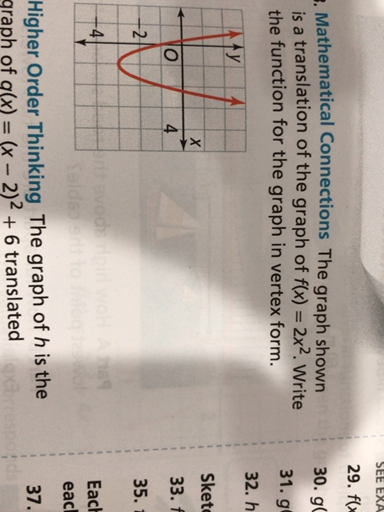Ask question

# To determine. The correct graph for the function[g(x)=-frac{1}{2}f(x)+1 is B# To determine. The correct graph for the function[g(x)=-frac{1}{2}f(x)+1 is B

Question
Transformation propertiesasked 2021-02-19
To determine.
The correct graph for the function$$\displaystyle{\left[{g{{\left({x}\right)}}}=-{\frac{{{1}}}{{{2}}}}{f{{\left({x}\right)}}}+{1}\right.}$$ is B

## Answers (1)2021-02-20

Vertical translation:
For $$\displaystyle{b}\ {>}\ {0}$$,
The graph of $$\displaystyle{y}={f{{\left({x}\right)}}}\ +\ {b}$$ is the graph of $$\displaystyle{y}={f{{\left({x}\right)}}}$$ {shifted up b units}
The graph of $$\displaystyle{y}={f{{\left({x}\right)}}}\ -\ {b}$$ is the graph of $$\displaystyle{y}={f{{\left({x}\right)}}}\ +\ {b}$$ {shifted down b units}
Reflection:
Across the x-axis:
The graph of $$\displaystyle{y}=\ -{f{{\left({x}\right)}}}$$ is the reflection of the graph of $$\displaystyle{y}={f{{\left({x}\right)}}}$$ across the x-axis
Across the y-axis:
The graph of $$\displaystyle{y}=\ {f{{\left(-{x}\right)}}}$$ is the reflection of the graph of $$\displaystyle{y}={f{{\left({x}\right)}}}$$ across the x-axis
Vertically Stretching or Shrinking:
The graph of $$\displaystyle{y}=\ {a}{f{{\left(-{x}\right)}}}$$ can be obtained from the graph of $$\displaystyle{y}={f{{\left({x}\right)}}}$$ by
Stretching verttically for $$\displaystyle{m}{i}{d}{a}{m}{i}{d}\ {>}\ {1}$$ or shrinking vertically for $$\displaystyle{a}\ {>}\ {m}{i}{d}{a}{m}{i}{d}\ {>}\ {1}$$.
For $$\displaystyle{a}{<}{0}$$, the graph is also reflected across the x-axis
. By the properties of transformation, the graph of $$\displaystyle{g{{\left({x}\right)}}}=-{\frac{{{1}}}{{{2}}}}{f{{\left({x}\right)}}}+{1}$$ is,
The transformation of $$\displaystyle{y}={f{{\left({x}\right)}}}$$ and shri
vertically by a factor of $$\displaystyle{\frac{{{1}}}{{{2}}}}$$
Then the graph is reflection about the x-axis and shifted up one unit.
From the given graphs, the graphs B satisfies the all condition's stated above.
Thus, the correct graph for the function $$\displaystyle{g{{\left({x}\right)}}}=-{\frac{{{1}}}{{{2}}}}{f{{\left({x}\right)}}}+{1}$$ is B

### Relevant Questionsasked 2021-05-16
Consider the curves in the first quadrant that have equationsy=Aexp(7x), where A is a positive constant. Different valuesof A give different curves. The curves form a family,F. Let P=(6,6). Let C be the number of the family Fthat goes through P.
A. Let y=f(x) be the equation of C. Find f(x).
B. Find the slope at P of the tangent to C.
C. A curve D is a perpendicular to C at P. What is the slope of thetangent to D at the point P?
D. Give a formula g(y) for the slope at (x,y) of the member of Fthat goes through (x,y). The formula should not involve A orx.
E. A curve which at each of its points is perpendicular to themember of the family F that goes through that point is called anorthogonal trajectory of F. Each orthogonal trajectory to Fsatisfies the differential equation dy/dx = -1/g(y), where g(y) isthe answer to part D.
Find a function of h(y) such that x=h(y) is the equation of theorthogonal trajectory to F that passes through the point P.asked 2021-03-20
The graph of y = f(x) contains the point (0,2), $$\displaystyle{\frac{{{\left.{d}{y}\right.}}}{{{\left.{d}{x}\right.}}}}={\frac{{-{x}}}{{{y}{e}^{{{x}^{{2}}}}}}}$$, and f(x) is greater than 0 for all x, then f(x)=
A) $$\displaystyle{3}+{e}^{{-{x}^{{2}}}}$$
B) $$\displaystyle\sqrt{{{3}}}+{e}^{{-{x}}}$$
C) $$\displaystyle{1}+{e}^{{-{x}}}$$
D) $$\displaystyle\sqrt{{{3}+{e}^{{-{x}^{{2}}}}}}$$
E) $$\displaystyle\sqrt{{{3}+{e}^{{{x}^{{2}}}}}}$$asked 2021-02-25
Provide answers to all tasks using the information provided.
a) Find the parent function f.
Given Information: $$g{{\left({x}\right)}}=-{2}{\left|{x}-{1}\right|}-{4}$$
b) Find the sequence of transformation from f to g.
Given information: $$f{{\left({x}\right)}}={\left[{x}\right]}$$
c) To sketch the graph of g.
Given information: $$g{{\left({x}\right)}}=-{2}{\left|{x}-{1}\right|}-{4}$$
d) To write g in terms of f.
Given information: $$g{{\left({x}\right)}}=-{2}{\left|{x}-{1}\right|}-{4}{\quad\text{and}\quad} f{{\left({x}\right)}}={\left[{x}\right]}$$asked 2021-02-08
a) Find the sequence of transformation from f to g
Given information: $$g{{\left({x}\right)}}=\frac{1}{{2}}{\left|{x}-{2}\right|}-{3}{\quad\text{and}\quad} f{{\left({x}\right)}}={x}^{3}$$
b) To sketch the graph of g.
Given information: $$f{{\left({x}\right)}}={\left|{x}\right|}$$
c) To write g in terms of f.
Given information: $$g{{\left({x}\right)}}=\frac{1}{{2}}{\left|{x}-{2}\right|}-{3}{\quad\text{and}\quad} f{{\left({x}\right)}}={\left|{x}\right|}$$asked 2021-05-05
The bulk density of soil is defined as the mass of dry solidsper unit bulk volume. A high bulk density implies a compact soilwith few pores. Bulk density is an important factor in influencing root development, seedling emergence, and aeration. Let X denotethe bulk density of Pima clay loam. Studies show that X is normally distributed with $$\displaystyle\mu={1.5}$$ and $$\displaystyle\sigma={0.2}\frac{{g}}{{c}}{m}^{{3}}$$.
(a) What is thedensity for X? Sketch a graph of the density function. Indicate onthis graph the probability that X lies between 1.1 and 1.9. Findthis probability.
(b) Find the probability that arandomly selected sample of Pima clay loam will have bulk densityless than $$\displaystyle{0.9}\frac{{g}}{{c}}{m}^{{3}}$$.
(c) Would you be surprised if a randomly selected sample of this type of soil has a bulkdensity in excess of $$\displaystyle{2.0}\frac{{g}}{{c}}{m}^{{3}}$$? Explain, based on theprobability of this occurring.
(d) What point has the property that only 10% of the soil samples have bulk density this high orhigher?
(e) What is the moment generating function for X?asked 2021-02-14
Mg reacts with H+ (aq) according to
$$\displaystyle{M}{g{{\left({s}\right)}}}+{2}{H}+{\left({a}{q}\right)}\to{M}{g}_{{2}}+{\left({a}{q}\right)}+{H}_{{2}}{\left({g}\right)}$$
Suppose that 0.524 g of Mg is reacted with 60.o ml of 1.0 M H+(aq). Assume that the density of the H+ (aq) solution os 1.00 g/ml,and that its specific heat capacity equals that of water. Theinitial and final temperatures are 22.0 degree celsius and 65.8degree celsius.
a) Is the reaction endothermic or exothermic?
b) Calculate $$\displaystyle\triangle{H}$$ of of th reaction. Use correct sigs andgive units.
c) Calculate the $$\displaystyle\triangle{H}$$ of the reaction per mole of magnesium.asked 2020-11-09
To determine:
To prove:
The function $$\displaystyle{T}:{V}\rightarrow{W}$$ is linear transformation if and only if
$$\displaystyle{T}{\left({a}{u}\ +\ {b}{v}\right)}={a}{T}{\left({u}\right)}\ +\ {b}{T}{\left({v}\right)}$$ for all vectors u and v and all scalars a and b.asked 2021-02-21The graph is a translation of the graph of f(x) = 2x². Write the function for the graph in vertex form.asked 2021-01-10
Whether T is a linear transformation, that is $$\displaystyle{T}:{C}{\left[{0},{1}\right]}\rightarrow{C}{\left[{0},{1}\right]}$$ defined by $$\displaystyle{T}{\left({f}\right)}={g}$$, where $$\displaystyle{g{{\left({x}\right)}}}={e}^{{{x}}}{f{{\left({x}\right)}}}$$asked 2021-03-12
Complete the tasks to determine:
a)To graf: The function $$g{{\left({x}\right)}}={2}^{{{x}-{4}}}.$$
b)The domain of the function $$g{{\left({x}\right)}}={2}^{{{x}-{4}}}$$ in the interval notation.
c)The range of the function $$g{{\left({x}\right)}}={2}^{{{x}-{4}}}$$ in the interval notation.
d)The equation of the asymptote of the function $$g{{\left({x}\right)}}={2}^{{{x}-{4}}}.$$
...